# Sort question in C++

Go to solution Solved by Danyfirex,

## Recommended Posts

Hi everybody,

I'm a total newbie in C++ . Just started it a couple of days ago using CodeBlocks IDE (which includes the MinGW compiler).
I found a script that gives a good sort result when the array is composed of integers.
But if I try to adapt it to doubles, then the sorting isn't accurate.

```/* qsort example */
#include <stdlib.h>     /* qsort */
#include <iostream>
using namespace std;

int values = { 40, 10, 100, 90, 20, 25, -50, -100, -1, 65 };

int compare (const void* a, const void* b) {
return ( *(int*)a - *(int*)b );
}

int main () {
qsort (values, 10, sizeof(int), compare);
for (int n=0; n<10; n++) {
cout << values[n] << endl;
}
return 0;
}```

Correct console result :

```-100
-50
-1
10
20
25
40
65
90
100```

Now let's try it for doubles :

```/* qsort example */
#include <stdlib.h>     /* qsort */
#include <iostream>
using namespace std;

double values = { -9.9995, 9.9995, -9.9997, 9.9997, -9.9996, 9.9996,
-9.9999, 9.9999, -9.9998, 9.9998 };

int compare (const void* a, const void* b) {
return ( *(double*)a - *(double*)b );
}

int main () {
qsort (values, 10, sizeof(double), compare);
for (int n=0; n<10; n++) {
cout << values[n] << endl;
}
return 0;
}```

Console result :

```-9.9995
-9.9999
-9.9997
-9.9996
-9.9998
9.9995
9.9999
9.9996
9.9997
9.9998```

Doubles aren't accurately sorted in the reworked script. Any idea what went wrong ?

Thanks

##### Share on other sites

I'd check the double-to-integer conversion you are doing in the compare function, floating math may not function like you'd expect.

An unrelated suggestion: You might wanna start with C first as it's the basis of C++ but it's a lot simpler in concept, nailing the fundamentals will really help you later in your C/C++ journeyEasyCodeIt - A cross-platform AutoIt implementation - Fund the development! (GitHub will double your donations for a limited time)

##### Share on other sites

Thanks for the quick answer, I'll try that##### Share on other sites
• Solution

```int compare(const void *x, const void *y)
{
double xx = *(double*)x, yy = *(double*)y;
if (xx < yy) return -1;
if (xx > yy) return  1;
return 0;
}```

Saludos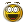Danysys.com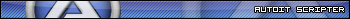AutoIt...

##### Share on other sites

@Danyfirex : it worked fine with your suggestion. . Now the console shows this :

```-9.9999
-9.9998
-9.9997
-9.9996
-9.9995
9.9995
9.9996
9.9997
9.9998
9.9999```

Guys, It's great to have you on this site##### Share on other sites

My journey through C++I just "created" a very short script in C++ for sorting a structure of integers, then compiled it into a dll and called it from AutoIt. Markyrocks did that in another thread a few days ago but I wanted to try all this by myself. Here is the result :

C++ code is 1 file only, named "main.cpp", here is its content :

```#include <stdlib.h>     /* qsort */

int comp (const void* x, const void* y) {
return *(int*)x - *(int*)y;
}

extern "C" void __declspec(dllexport) my_sort(int* my_struct, int nb_elem) {
qsort(my_struct, nb_elem, sizeof(int), comp);
}```

AutoIt code for testing  :

```#include <WinAPIDiag.au3>

\$iNb_elem = 10000 ; change this value for testing less or more elements

\$hDLL  = DllOpen(@ScriptDir & "\Structure 03.dll")
If \$hDLL = -1 Then Exit Msgbox(0, "DllOpen", "error occured")

_SplashOn("Filling structure")
\$tStruct = DllStructCreate("int arr[" & \$iNb_elem & "]")
For \$i = 1 To \$iNb_elem
\$tStruct.arr((\$i)) = Random(-100000, +100000, 1) ; note ((\$i)) and not (\$i) <=================
Next
SplashOff()

If \$iNb_elem <= 10000 Then
_SplashOn("Displaying structure #1")
_WinAPI_DisplayStruct(\$tStruct, "int arr[" & \$iNb_elem & "]", "\$tStruct UNsorted")
SplashOff()
EndIf

\$hTimer = TimerInit()
\$aRet = DllCall(\$hDLL, "none:cdecl", "my_sort", "ptr", DllStructGetPtr(\$tStruct), "int", \$iNb_elem)
If @error Then Exit Msgbox(0, "DllCall", "error " & @error & " occured")

If IsArray(\$aRet) Then
ConsoleWrite(\$iNb_elem & " elements sorted in " & TimerDiff(\$htimer) & " ms" & @CRLF)
If \$iNb_elem <= 10000 Then
_SplashOn("Displaying structure #2")
_WinAPI_DisplayStruct(\$tStruct, "int arr[" & \$iNb_elem & "]", "\$tStruct sorted")
SplashOff()
EndIf
Else
Exit Msgbox(0, "error", "\$aRet is not an Array")
EndIf

DllClose(\$hDLL)

;==========================================================
Func _SplashOn(\$sFirstLine, \$sSecondLine = "please wait...")
SplashTextOn("", \$sFirstLine & @CRLF & \$sSecondLine, _
250, 50, -1, -1, \$DLG_NOTITLE + \$DLG_TEXTVCENTER)
EndFunc   ;==>_SplashOn```

Results (with 10 elements only (for pics convenience) :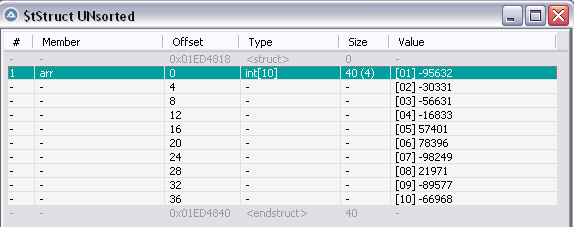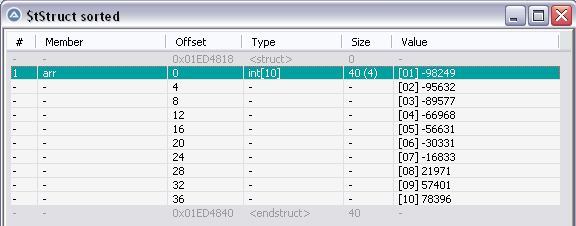Notes : the AutoIt variable \$iNb_elem contains the number of elements for the test.
* Until 10.000 elements, it will sort then display the results in the _WinAPI_DisplayStruct() GUI as shown in the above pics
* If > 10.000 elements, it will sort but won't display anything (because it may take too long to display). This 10.000 value for displaying can be easily changed in the script)

In all cases, the time for sorting will show in AutoIt console. For example, on my old PC, it sorts 1 million elements in less than 1s

AutoIt Console :

`1000000 elements sorted in 493.493167427704 ms`

I have no idea if I should attach the dll or not because VirusTotal indicates that 1AV (out of 66 !) flags the dll malicious (this AV is named ESET-NOD32) . Anyway, you got the C++ code so you can compile it by yourself if interested, in case you have the appropriate tool for that (I used the MinGW compiler found in CodeBlocks IDE)

My next step is to rework the Dll to allow float & doubles sorting too. If you guys have comments on the C++ code above, please don't hesitate to comment as I'm a total beginner in C++

Mods : could you please be kind enough and suppress my precedent deleted message ?

ThanksEdited by pixelsearch
shortened C++ code
##### Share on other sites

@pixelsearch I try to write your C++ code in Freebasic.
To run the code you can use this site https://www.jdoodle.com/execute-freebasic-online/

1st time I am coding using Freebasic so getting warning, may be @UEZ can point out my mistake.
warning 3(2): Passing different pointer types...

```#include "crt/stdlib.bi"

Declare Function QCompare cdecl (ByVal e1 As Any Ptr, ByVal e2 As Any Ptr) As Integer

Sub PrintArray( karray() As Integer )
For position As Integer = LBound(karray) To UBound(karray)
Print karray(position) ;
Next
Print  !"\n";
End Sub

Function QCompare cdecl (ByVal e1 As Any Ptr, ByVal e2 As Any Ptr) As Integer
Return (*(CPtr(Integer Ptr, e1)) - *(CPtr(Integer Ptr, e2)))
End Function

Dim oarray(9) As Integer = { 10, 9, 6, 8, 5, 1, 3, 2, 7, 4 }

PrintArray( oarray() )
qsort @oarray(0), 10, Sizeof(Integer), @QCompare
PrintArray( oarray() )```

```Sub PrintArray( karray() As Integer )
For position As Integer = LBound(karray) To UBound(karray)
Print karray(position) ;
Next
Print  !"\n";
End Sub

Function Partition( Array() As Integer, start As Integer, oEnd As Integer) As Integer
Dim pivot as Integer
Dim t as Integer
Dim As Integer i, j

pivot = Array(oEnd)
i = (start - 1)
For j = start To oEnd - 1
If (Array(j) < pivot) Then
i = i + 1
t = Array(i)
Array(i) = Array(j)
Array(j) = t
End If
Next
t = Array(i + 1)
Array(i+1) = Array(oEnd)
Array(oEnd) = t
Partition = (i + 1)
End Function

Sub quickSort( Array() As Integer, start As Integer, oEnd As Integer)
Dim p As Integer
If (start < oEnd) Then
p = Partition(Array(), start, oEnd)
quickSort(Array(), start, p - 1)
quickSort(Array(), p + 1, oEnd)
End If
End Sub

Dim oarray(9) As Integer = { 10, 9, 6, 8, 5, 1, 3, 2, 7, 4 }

PrintArray( oarray() )
quickSort( oarray(), 0, UBound(oarray))
PrintArray( oarray() )```

##### Share on other sites

1st time I am coding using Freebasic so getting warning, may be @UEZ can point out my mistake.
warning 3(2): Passing different pointer types...

You have to cast the last parameter (pointer to function):

`qsort @oarray(0), 10, Sizeof(Integer), Cast(Any Ptr, @QCompare)`

Or use Quicksort written in FB:

```Sub QsortA(array() As Integer, _start As Integer, _end As Uinteger) 'ascending
_end = Iif(_end > Ubound(array), Ubound(array), _end)
_start = Iif(_start < 0, 0, _start)
Dim As Integer I = _start, J = _end
Dim As Integer X = array(((I + J) \ 2))
While I <= J
While array(I) < X  'change to > for descending
I += 1
Wend
While array(J) > X  'change to < for descending
J -= 1
Wend
If I <= J Then
Swap array(I), array(J)
I += 1
J -= 1
Endif
Wend
If J > _start Then QsortA(array(), _start, J)
If I < _end Then QsortA(array(), I, _end)
End Sub

Sub PrintArray( karray() As Integer )
For position As Integer = LBound(karray) To UBound(karray)
Print karray(position) ;
Next
Print  !"\n";
End Sub

Dim oarray(9) As Integer = { 10, 9, 6, 8, 5, 1, 3, 2, 7, 4 }

PrintArray( oarray() )
QsortA( oarray(), 0, Ubound(oarray))
PrintArray( oarray() )

Sleep```

Edited by UEZ

The own fart smells best!
Her 'sikim hıyar' diyene bir avuç tuz alıp koşma!
¯\_(ツ)_/¯  ٩(●̮̮̃•̃)۶ ٩(-̮̮̃-̃)۶ૐ

##### Share on other sites

@UEZ Thanks##### Share on other sites

I got no issues with this.  Would have been nice to get a tag.  Pixelsearch seems like one of the good ones.  If anyone was following the other thread as I said in there the new version is drastically different than this.  The whole thing was essentially rewritten to get rid of any copying except when absolutely necessary (strings) .  That being said it doesn't even need a struct it just sorts the pointers at the actual autoit variant type variable.   That and I eliminated all calls to processread/write.  I'm almost ready to drop a new version.   Getting to the strings is some Uber obscure syntax but I got em.  I promise I'm not going to hijack this thread.  I would have helped out but I didn't see this until just now.  It took me roughly 5 years to get to this point with c++.  I'm like so good with it I'm beginning to think that I can see into the future.   Joking.  Goodluck.  Take care.

##### Share on other sites
• Developers
2 hours ago, markyrocks said:

If anyone was following the other thread as I said in there the new version is drastically different than this.  The whole thing was essentially rewritten to get rid of any copying except when absolutely necessary (strings) .

You can't leave it alone can you and even listen to a simple request,  so let me make it very and ultimately simple for you:

Consider this your final warning. Post one other comment like this and you will be removed from our forums.

Jos

Edited by Jos

Live for the present,
Dream of the future,
Learn from the past.##### Share on other sites
• 2 weeks later...

Hi everybody
A few days ago, I was lucky to discover in this link a thread from @funkey (dated from 2013) where we can find a part in AutoIt and a part in C. funkey's script is interesting, not only for its content, but it helps to learn C and C++ when you're a newbie like me, as it's nicely written.

As it was challenging, then I tried to write some simple code to quickly sort a 1D array of strings (using a dll) and the results look promisingFirst of all, here is my code in AutoIt :

```#include <Array.au3>

\$hDLL = DllOpen(@ScriptDir & "\rrr.dll")
If \$hDLL = -1 Then Exit Msgbox(0, "DllOpen", "error occured")

;====================
;~ Local \$aArray = ["john", "bobby", "hello", "test", "catherine", "nomi", "shinta", _
;~                     "martin", "abe", "may", "zeno", "zack", "angel", "gaby"] ; 56 + 14 delim => 80

;~ ; OR for more elements :
Local \$iItems = 100000, \$aArray[\$iItems]
FillArray(\$aArray, \$iItems)

_ArrayDisplay(\$aArray, "UNsorted")

;====================
\$hTimer = TimerInit()

Local \$iRows = Ubound(\$aArray), \$sDelim = Chr(1), \$sData = ""

For \$i = 0 To \$iRows - 1
\$sData &= \$aArray[\$i] & \$sDelim
Next

ConsoleWrite("Preparing concatenated string = " & Int(TimerDiff(\$htimer)) & " ms" & @crlf)

;====================
\$hTimer = TimerInit()

\$iBufferSize = StringLen(\$sData)
\$tStruct = DllStructCreate("char[" & \$iBufferSize & "]")
If @error Then Exit Msgbox(0, "DllStructCreate", "error " & @error)

DllStructSetData(\$tStruct, 1, \$sData)
If @error Then Exit Msgbox(0, "DllStructSetData", "error " & @error)

ConsoleWrite("Writing structure = " & Int(TimerDiff(\$htimer)) & " ms" & @crlf)

MsgBox(0, "Pointer of struct", DllStructGetPtr(\$tStruct) & "   Buffer size = " & \$iBufferSize)

;====================
\$hTimer = TimerInit()

\$aRet = DllCall(\$hDLL, "int:cdecl", "StringSort", "ptr", DllStructGetPtr(\$tStruct), "str", \$sDelim, "int", \$iRows)
If @error Then Exit Msgbox(0, "DllCall StringSort", "error " & @error)

ConsoleWrite("DllCall = " & Int(TimerDiff(\$htimer)) & " ms" & @crlf)

_ArrayDisplay(\$aRet, "\$aRet")

;====================
\$hTimer = TimerInit()

\$sSorted = DllStructGetData(\$tStruct, 1)
If @error Then Exit Msgbox(0, "DllStructGetData", "error " & @error)

ConsoleWrite("Reading structure = " & Int(TimerDiff(\$htimer)) & " ms" & @crlf)

;====================
\$hTimer = TimerInit()

Local \$aArray2 = StringSplit(\$sSorted, Chr(1), 2) ;  \$STR_NOCOUNT = 2

ConsoleWrite("Generating sorted 1D array = " & Int(TimerDiff(\$htimer)) & " ms" & @crlf)

_ArrayDisplay(\$aArray2, "Sorted")

_StringSplitFree(\$tStruct)

DllClose(\$hDLL)

;======================================
Func FillArray( ByRef \$aItems, \$iItems ) ; LarsJ (fill array with random strings)
Local \$aLetters = [ 'a', 'b', 'c', 'd', 'e', 'f', 'g', 'h', 'i', 'j', 'k', 'l', 'm', _
'n', 'o', 'p', 'q', 'r', 's', 't', 'u', 'v', 'w', 'x', 'y', 'z' ], \$s
For \$i = 0 To \$iItems - 1
\$s = \$aLetters[Random(0,25,1)]
For \$j = 1 To Random(10,30,1)
\$s &= \$aLetters[Random(0,25,1)]
Next
\$aItems[\$i] = \$s
Next
EndFunc   ;==>_FillArray

;======================================
Func _StringSplitFree(ByRef \$tStruct) ; Funkey
DllCall("msvcrt.dll", "none:cdecl", "free", "ptr", DllStructGetPtr(\$tStruct))
If @error Then Exit Msgbox(0, "DllCall Free", "error " & @error & " occured")
\$tStruct = 0
EndFunc   ;==>_StringSplitFree```

Now the  C++ code :

```#include <algorithm>     // sort
#include <string.h>      // strtok, strcpy
#include <string>        // string
using namespace std;

extern "C" __declspec(dllexport) int StringSort(char* structure, char* sdelim, int irows)
{
string arr[irows];
int i = 0, ipos = 0;
char* p;

p = strtok(structure, sdelim); // split string into tokens (returns a pointer).
// End of the token (delimiter) is automatically replaced by a null-character
// https://www.cplusplus.com/reference/cstring/strtok/
while ((p != NULL) && (i < irows))
{
arr[i] = p;
p = strtok(NULL, sdelim);
i++;
}

sort(arr, arr + irows);

for(i = 0; i < irows; ++i)
{
strcpy(&structure[ipos], (arr[i] +
((i < irows - 1) ? sdelim : "")).c_str()); // strcpy adds a x00 at the end

ipos += arr[i].size() + 1; // overwrite each preceding x00 from strcpy
}

return 0;
}```

The result on 100.000 random strings looks great on my antique computer. This is what AutoIt console shows for 100.000 strings (about 2s for the whole process) :

```Preparing concatenated string = 651 ms
Writing structure = 44 ms
DllCall = 770 ms
Generating sorted 1D array = 542 ms```

Here are the different phases I went through, based on a simpler array of 14 strings (also found in the AutoIt code) :

* create a string composed of all the (unsorted) array elements, separated by a delimiter. I choosed chr(1) as delimiter, maybe we'll discuss this point later in case you guys have a better idea. There's also a delimiter chr(1) at the end of the concatenated string.

* Still in AutoIt, create a structure of char's and populate it with a single instruction (no loop) . Here is what the corresponding memory dump shows at this exact moment :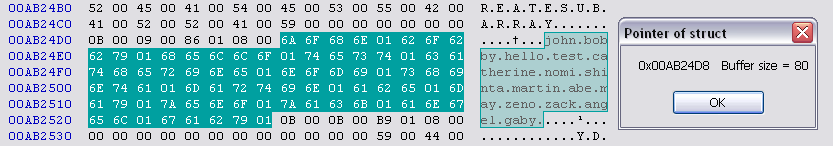* DllCall : this phase will :

a) Split the big unsorted string found in the structure, ending with tokens (each token is an element of a new C array) . It's the C strtok instruction that splits and create tokens.
b) Then Sort the Array of strings using C Sort
c) Finally overwrite the structure using C strcpy instruction, overwriting carefully each chr(0) that strtok added (it replaced all my separators chr(1) with chr(0) !) . So we'll replace all chr(0) with the originals chr(1), except for the very last one.
Watch out carefully for the last byte of the buffer, to avoid ending with a "buffer overflow"... only for one last byte !
Here is what the corresponding memory dump shows at this exact moment : the strings are sorted and there's no buffer overflow if you look at the 81th byte which is outside the buffer (its content is still 0B as in the preceding pic) :* Back to AutoIt : now we read the structure with 1 instruction (no loop) and StringSplit is used to immediately create a new sorted array, that's it.

I compiled the C/C++ code with the the free MinGW compiler found in CodeBlocks 17.12 IDE . In case you're interested, it should be easy for you to do same.

Thanks for readingEdit 28 january 2022 :
Allocate memory on heap to avoid a possible stack overflow (error 0xC00000FD) if too many elements in the array, by doing what follows in C++ code. Replace this :

`string arr[irows];`

With that :

```// dynamic memory allocation for the array :
string *arr = new string[irows]; // works with 1.000.000 elements

...

// before returning, delete the dynamically allocated memory
delete [] arr;```

It seems that vectors could have been used instead, but I'm not familiar with them until now. One day maybe...

Next step should be to work on 2D arrays. I'll create a new post if the results are promising,

Edit 10 february 2022 :
Though the preceding script works fine, it will soon be replaced with C++ code based on a Vector of structs (in a future post of this thread)

Edited by pixelsearch
##### Share on other sites

Hi everybody,
After reading a bit about Vectors in C++, I could quickly sort a string column in a 2D array (example made with an AutoIt 2D array of 100.000 rows and 6 cols, also tried 500.000 rows). Here are the corresponding codes :

AutoIt code :

```#include <Array.au3>
; #include <WinAPIDiag.au3>
#include "RandomArray.au3" ; LarsJ

\$hDLL = DllOpen(@ScriptDir & "\rrr_03.dll")
If \$hDLL = -1 Then Exit Msgbox(0, "DllOpen", "error occured")

Global \$g_iRows = 100000, \$g_iCols = 6, \$g_iSort_Col = 0, \$g_aArray ; only col 0 is string

_Generate_All(\$g_aArray)
Local \$aBackup = \$g_aArray ; extremely fast !

_ArrayDisplay(\$g_aArray, "UNsorted", Default, Default, Default, _
"Strings|Integers*|Floats*|Dates*|Times*|R/C*")

;====================
\$hTimer = TimerInit()

Local \$sDelim = Chr(1), \$sData = ""

For \$i = 0 To \$g_iRows - 1
\$sData &= \$g_aArray[\$i][\$g_iSort_Col] & \$sDelim
Next

ConsoleWrite("Preparing concatenated string = " & Int(TimerDiff(\$htimer)) & " ms" & @crlf)

;====================
\$hTimer = TimerInit()

\$iBufferSize = StringLen(\$sData)
\$tStruct = DllStructCreate("char[" & \$iBufferSize & "]")
If @error Then Exit Msgbox(0, "DllStructCreate", "error " & @error)

DllStructSetData(\$tStruct, 1, \$sData)
If @error Then Exit Msgbox(0, "DllStructSetData", "error " & @error)

ConsoleWrite("Writing structure = " & Int(TimerDiff(\$htimer)) & " ms" & @crlf)

MsgBox(0, "Pointer of struct", DllStructGetPtr(\$tStruct) & "   Buffer size = " & \$iBufferSize)

\$tStruct2 = DllStructCreate("int[" & \$g_iRows & "]") ; will be filled by dll
If @error Then Exit Msgbox(0, "DllStructCreate 2", "error " & @error)

;====================
\$hTimer = TimerInit()

\$aRet = DllCall(\$hDLL, "int:cdecl", "StringSort2D", "ptr", DllStructGetPtr(\$tStruct), _
"ptr", DllStructGetPtr(\$tStruct2), "str", \$sDelim, "int", \$g_iRows)
If @error Then Exit Msgbox(0, "DllCall StringSort2D", "error " & @error)

ConsoleWrite("DllCall = " & Int(TimerDiff(\$htimer)) & " ms" & @crlf)

_ArrayDisplay(\$aRet, "\$aRet")
; _WinAPI_DisplayStruct(\$tStruct2, "int[" & \$g_iRows & "]", "\$tStruct2")

;====================
\$hTimer = TimerInit()

For \$i = 0 To \$g_iRows - 1
\$iIndex = DllStructGetData(\$tStruct2, 1, \$i + 1) ; row index before sorting
If \$i <> \$iIndex Then
For \$j = 0 To \$g_iCols - 1
\$g_aArray[\$i][\$j] = \$aBackup[\$iIndex][\$j]
Next
EndIf
Next

ConsoleWrite("Generating sorted 2D array = " & Int(TimerDiff(\$htimer)) & " ms" & @crlf)

_ArrayDisplay(\$g_aArray, "sorted (col " & \$g_iSort_Col & ")", Default, Default, Default, _
"Strings|Integers*|Floats*|Dates*|Times*|R/C*")

_StringSplitFree(\$tStruct)
_StringSplitFree(\$tStruct2)
DllClose(\$hDLL)

;=============================================
Func _Generate_All(ByRef \$g_aArray) ; LarsJ
ConsoleWrite("\$g_iRows = " & \$g_iRows & "    \$g_iCols = " & \$g_iCols & @CRLF)
\$g_aArray = FAS_Random2DArrayAu3(\$g_iRows, "sifdtr", "abcdefghijklmnopqrstuvwxyz")
EndFunc   ;==>_Generate_All

;=============================================
Func _StringSplitFree(ByRef \$tStruct) ; Funkey
DllCall("msvcrt.dll", "none:cdecl", "free", "ptr", DllStructGetPtr(\$tStruct))
If @error Then Exit Msgbox(0, "DllCall Free", "error " & @error & " occured")
\$tStruct = 0
EndFunc   ;==>_StringSplitFree```

C++ code

```// build options OF THE PROJECT : check line C++1z (C++17) or these errors appear :
// error: 'to_string' was not declared in this scope
// error: 'stoi' was not declared in this scope

#include <algorithm>    // sort
#include <string.h>     // strtok
#include <string>       // string
#include <vector>       // vector
using namespace std;

bool sortcol(const vector<string>& v1, const vector<string>& v2)
{
return v1 < v2; //  sort on col 0,  on col 1 etc... (not in this dll)

// To sort descending :
// return v1 > v2;
}

extern "C" __declspec(dllexport) int StringSort2D(char* structure, int* structure2, char* sdelim, int irows)
{
vector<vector<string> > vect(irows, vector<string>(2)); // irows and 2 cols

int i = 0;
char* p;

p = strtok(structure, sdelim); // split string into tokens (returns a pointer).
// End of the token (delimiter) is automatically replaced by a null-character
// https://www.cplusplus.com/reference/cstring/strtok/

while ((p != NULL) && (i < irows))
{
vect[i] = p; // string (col 0 will be sorted)
vect[i] = to_string(i); // row (to remember initial index before sorting)
p = strtok(NULL, sdelim);
i++;
}

sort(vect.begin(), vect.end(), sortcol); // sort on column 0 (string) in this dll

for(i = 0; i < irows; ++i)
{
*(structure2 + i) = stoi(vect[i]); // index of sorted string [i] ... before sorting
}

// this should release memory used by the vector (to be confirmed) :
// https://en.wikibooks.org/wiki/More_C%2B%2B_Idioms/Clear-and-minimize
// http://www.cplusplus.com/reference/vector/vector/clear/ <= recommeneds swap()
// http://www.cplusplus.com/reference/vector/vector/swap-free/
vector<vector<string>>().swap(vect);

return 0;
}```

I kept a part of  the flowchart used for 1D array (see precedent post) but had to adapt it for 2D, by adding this :

* Create a 2nd structure (int) in AutoIt to be filled by the Dll during DllCall(). This structure will contain the index (row) of each sorted string ... before it was sorted. Here is an example based on 10 strings :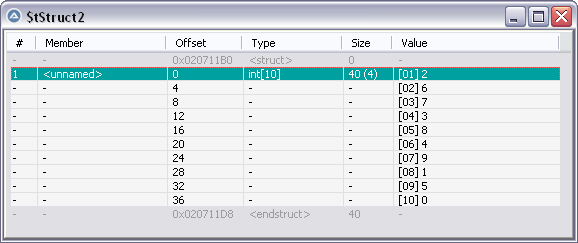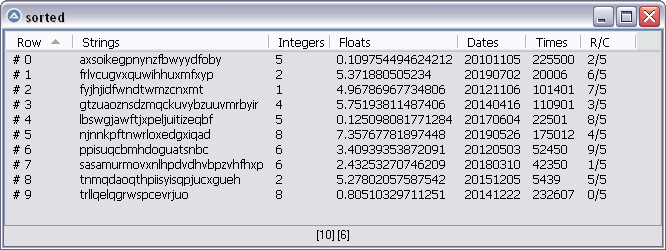As you can see in the structure pic (the 2nd pic with its 1st line in green) the value of the 1st line in the structure is 2. It means that the 1st string sorted ("axso..." in the sorted pic) HAD an index of 2 in the unsorted pic.

This goes for all the other sorted strings. Same explanation for another string : the last string sorted ("trll..." in the sorted pic) HAD an index of 0 in the unsorted pic.

This index in the structure will be used to grab the correct values of all the other columns in a backup of the initial array (\$aBackup), pointing to the correct row.

So in the C++ code, the 2D vector has only 2 columns :
- a column for the unsorted strings
- a column for the index (row) of the string in the Autoit array (which is 0, 1, 2, 3... before the sort)

After the sort is done on the string column (in the Dll), the strings will be sorted in the 1st column and their "index before sorting" will be found unaltered in the other column, which will fill the index structure. There are certainly other ways to achieve this but this is what I found.

The next step should be to create a udf to take care of all cases (1D, 2D, col to be sorted, numeric or alphabetic sort, asc or desc) and to separate functions in the dll (all sorting functions should be based on vectors in the Dll, even if it's a 1D array in AutoIt)

If something is ready one day, I'll post it here. Meanwhile, I hope the code will be helpful for some users.

The hardest part I faced ?
Find the C++ code to declare a 2D vector of strings as "easily" as we declare a 2D array in AutoIt`vector<vector<string> > vect(irows, vector<string>(2)); // irows and 2 cols`

Edit 10 february 2022 :
Though the preceding script works fine, it will soon be replaced with C++ code based on a Vector of structs (in a future post of this thread)

Edited by pixelsearch
As the original array is 2D, return only the indexes from the dll, not the sorted data like in the preceding 1D example
##### Share on other sites

Thank you guys for your appreciations !

@jpm : what follows is for information.
I just tried the preceding code... in ArrayDisplayInternals beta (which uses a virtual listview and "columns indexes" stored in arrays). Please note that my reworked version of ArrayDisplayInternals beta doesn't prepare any column index when launched (except of course the mandatory "fast as light"index of the "Row" column).

Using the preceding AutoIt code & dll  to prepare the index of a string column in ArrayDisplay runs 10 times faster than the beta, so sky could be the limit, not only to quickly display the virtual LV, but also to rapidly prepare any column index when the user clicks on a column header to sort the column.

This is how I tested it on the 2D array of 100.000 rows and 6 cols :
* Replaced all code found inside this function (which returned \$tIndex) ...

```Func __ArrayDisplay_SortArrayStruct(Const ByRef \$aArray, \$iCol)
...
Return \$tIndex
EndFunc```

...with these lines of code inside the same function (which returns the equivalent \$tStruct2)

```Static \$hDLLSort = DllOpen(@ScriptDir & "\rrr_03.dll")
If \$hDLLSort = -1 Then Exit Msgbox(\$MB_TOPMOST, "DllOpen", "error occured")

Local \$sDelim = Chr(1), \$sData = ""
For \$i = 0 To \$_g_ArrayDisplay_nRows - 1
\$sData &= \$aArray[\$i][\$iCol] & \$sDelim ; delimiter also at the end of the string
Next

Local \$iBufferSize = StringLen(\$sData)
Local \$tStruct = DllStructCreate("char[" & \$iBufferSize & "]")
DllStructSetData(\$tStruct, 1, \$sData)

Local \$tStruct2 = DllStructCreate("int[" & \$_g_ArrayDisplay_nRows & "]")

Local \$aRet = DllCall(\$hDLLSort, "int:cdecl", "StringSort2D", "ptr", DllStructGetPtr(\$tStruct), _
"ptr", DllStructGetPtr(\$tStruct2), "str", \$sDelim, "int", \$_g_ArrayDisplay_nRows)

If @error Then Exit Msgbox(\$MB_TOPMOST, "DllCall StringSort2D", "error " & @error)

Return \$tStruct2```

* Placed the rrr_03.dll (what a name...) in the script directory

And the timers spoke for themselves : after a single click on the Strings column header (to sort it ascending, i.e to prepare its index) : 10 times faster with the dll compared to the beta.

This test was done to let you (and other readers) know that it is possible, for a user who needs it, to have a super sorting speed on columns in ArrayDisplay beta (or in any script involving virtual listviews). That could be useful when plenty of rows are involved.

The only thing I'll have to check is : can we do a natural sort in C++ ?
Because the C++ code I provided in the preceding post doesn't reflect it.

Also, this code would require to indicate what kind of sort is needed (strings, integers, float etc...) to run the appropriate C++ code, which could be a burden for users. Of course several functions could be found in the dll, (1D or 2D vectors), <string> <int> or <double> etc...

Jpm, all this is purely informative and it's not to ask you to modify anything in ArrayDisplayInternals beta, but I had to share this test with you, because you (and @LarsJwho provided the initial idea to use a virtual listview with column indexes) were totally involved in the rewriting of ArrayDisplayInternals.

To conclude, let me say that I'm really happy to use ArrayDisplayInternals beta because it displays the listview at the speed of light in any script. Also, how could have I ever done all the tests of the preceding posts (which involve plenty of rows) without it ?

Thanks for reading and stay healthyEdited by pixelsearch
16 feb 2022 : the code in this post is no more accurate (it has been improved since), anyway it was just to share my point of view with jpm
##### Share on other sites

Dll method is faster but still can you do speed test to see how much it's slower than your dll method (on AutoIt 2D array of 100000 rows and 6 cols)?
Can do the test myself as don't know how to compiled C++ code.

```#include <Array.au3>

__Example1()
Func __Example1()
Local \$arry[][] = [['D0',4,'D2'],['F0',6,'F2'],['A0',1,'A2'],['E0',5,'E2'],['C0',3,'C2'],['B0',2,'B2']]
_ArrayDisplay(\$arry)

;Local \$hTimer1 = TimerInit()
Local \$x_Result = __ArraySortJs(\$arry, 1)
;ConsoleWrite("time = " & TimerDiff(\$hTimer1) & @CRLF)
_ArrayDisplay(\$x_Result)
EndFunc

; #FUNCTION# =============================================================================
; Name...........: __ArraySortJs
; ========================================================================================
Func __ArraySortJs(\$o_array, \$o_Column = 0, \$o_Numeric = True, \$o_ascending = True)
;====
If Not IsArray(\$o_array) Then Return SetError(1, 0, -1)
If (UBound(\$o_array, 2) = 1) And (\$o_Column > 0) Then Return SetError(1, 0, -1)
If (UBound(\$o_array, 2) > 1) And (\$o_Column > UBound(\$o_array, 2) - 1) Then Return SetError(1, 0, -1)
;====

;====
Local \$o_CBlock = 'function GetArray(arr){' & @CRLF & _
'var oArray = new VBArray(arr)' & @CRLF & _
'return oArray.toArray()' & @CRLF & _
'}' & @CRLF & _
@CRLF & _
'function NumSort(a, b){' & @CRLF & _
'if (a === b) {' & @CRLF & _
'return 0' & @CRLF & _
'}' & @CRLF & _
'else {' & @CRLF & _
'return (a < b) ? -1 : 1' & @CRLF & _
'}' & @CRLF & _
'}' & @CRLF & _
@CRLF & _
'function ArraySorting(arr, oNumeric, oascending){' & @CRLF & _
'var JsArray = GetArray(arr)' & @CRLF & _
'if (oNumeric) {' & @CRLF & _
'JsArray.sort(NumSort)' & @CRLF & _
'}' & @CRLF & _
'else {' & @CRLF & _
'JsArray.sort()' & @CRLF & _
'}' & @CRLF & _
'if (oascending) {' & @CRLF & _
'return JsArray.toString()' & @CRLF & _
'}' & @CRLF & _
'else {' & @CRLF & _
'return JsArray.reverse().toString()' & @CRLF & _
'}' & @CRLF & _
'}'
;====

;====
Local \$ObjErr = ObjEvent("AutoIt.Error", "_ErrorHandler")
Local \$o_Obj = 0
\$o_Obj = ObjCreate("ScriptControl")
\$o_Obj.Language = "JScript"
;====

;====
If UBound(\$o_array, 2) = 0 Then
Local \$o_SortData = \$o_Obj.run("ArraySorting" , \$o_array, \$o_Numeric, \$o_ascending)
\$o_Obj = 0

Local \$o_SortArry = StringSplit(\$o_SortData, ',', 2)
Return \$o_SortArry
EndIf
;====

;====
Local \$o_ExtColmn[UBound(\$o_array)]
For \$i = 0 To UBound(\$o_array) - 1
\$o_ExtColmn[\$i] = \$o_array[\$i][\$o_Column]
Next

Local \$o_SortData = \$o_Obj.run("ArraySorting" , \$o_ExtColmn, \$o_Numeric, \$o_ascending)
\$o_Obj = 0
;====

;====
Local \$o_SortArry = StringSplit(\$o_SortData, ',', 2)

Local \$o_TempStr = ''
Local \$o_Index[Ubound(\$o_array)][Ubound(\$o_array, 2)]
For \$i = 0 To Ubound(\$o_SortArry) - 1
For \$j = 0 To Ubound(\$o_ExtColmn) - 1
If (\$o_SortArry[\$i] = \$o_ExtColmn[\$j]) AND Not StringRegExp(\$o_TempStr, '\b'& \$j &'\b') Then
\$o_TempStr &= \$j & '|'
For \$k = 0 To Ubound(\$o_array, 2) - 1
\$o_Index[\$i][\$k] = \$o_array[\$j][\$k]
Next
ExitLoop(1)
Endif
Next
Next
Return \$o_Index
;====
EndFunc

Func _ErrorHandler(\$oError)
EndFunc```

##### Share on other sites
On 2/1/2022 at 1:32 PM, jugador said:

Can you do speed test to see how much it's slower than your dll method (on AutoIt 2D array of 100000 rows and 6 cols)?

Yes we canUnfortunately, the Jscript function you provided in your last post gave bad timers on my antique computer.

Please everybody, be nice and don't ask why I'm not changing my computer for a more recent one. I don't want to start a debate on the usefulness of doing it when the end of life is nearly knocking at my door, thank you.

Back to your script, this is how I tested it, after commenting your __Example1() and adding an __Example2() + Func _Generate_All()

```#cs
#include <Array.au3>

__Example1()
Func __Example1()
; Local \$arry[][] = [['D0',4,'D2'],['F0',6,'F2'],['A0',1,'A2'],['E0',5,'E2'],['C0',3,'C2'],['B0',2,'B2']]
Local \$arry[][] = [['D0',6,'DD2'],['F0',4,'FF2'],['A0',1,'A2'],['E0',5,'E2'],['C0',3,'C2'],['B0',2,'B2']]
_ArrayDisplay(\$arry, "UNsorted")

Local \$hTimer1 = TimerInit()
; Local \$x_Result = __ArraySortJs(\$arry, 1) ; sort on col 1 (Jugador)
Local \$x_Result = __ArraySortJs(\$arry, 0, False) ; sort on col 0, not numeric
If @error Then Exit Msgbox(0, "__ArraySortJs", "error " & @error)
ConsoleWrite("time = " & TimerDiff(\$hTimer1) & @CRLF)
_ArrayDisplay(\$x_Result, "sorted")
EndFunc
#ce

#include <Array.au3>
#include "RandomArray.au3" ; LarsJ
Opt("MustDeclareVars", 1)

Global \$g_iRows = 1000, \$g_iCols = 6, \$g_aArray

__Example2()
Func __Example2()
_Generate_All(\$g_aArray)
_ArrayDisplay(\$g_aArray, "UNsorted", Default, Default, Default, _
"Strings|Integers*|Floats*|Dates*|Times*|R/C*")

Local \$hTimer2 = TimerInit()
Local \$x_Result2 = __ArraySortJs(\$g_aArray, 0, False) ; sort on col 0, not numeric
If @error Then Exit Msgbox(0, "__ArraySortJs", "error " & @error)
ConsoleWrite("JScript: sorted in = " & Int(TimerDiff(\$htimer2)) & " ms" & @crlf)
_ArrayDisplay(\$x_Result2, "sorted", Default, Default, Default, _
"Strings|Integers*|Floats*|Dates*|Times*|R/C*")
EndFunc

;===========================================
Func _Generate_All(ByRef \$g_aArray) ; LarsJ
ConsoleWrite("\$g_iRows = " & \$g_iRows & "    \$g_iCols = " & \$g_iCols & @CRLF)
\$g_aArray = FAS_Random2DArrayAu3(\$g_iRows, "sifdtr", "abcdefghijklmnopqrstuvwxyz")
EndFunc   ;==>_Generate_All

; #FUNCTION# =============================================================================
; Name...........: __ArraySortJs
; ========================================================================================
Func __ArraySortJs(\$o_array, \$o_Column = 0, \$o_Numeric = True, \$o_ascending = True)
;====
If Not IsArray(\$o_array) Then Return SetError(1, 0, -1)
If (UBound(\$o_array, 2) = 1) And (\$o_Column > 0) Then Return SetError(1, 0, -1)
If (UBound(\$o_array, 2) > 1) And (\$o_Column > UBound(\$o_array, 2) - 1) Then Return SetError(1, 0, -1)
;====

;====
Local \$o_CBlock = 'function GetArray(arr){' & @CRLF & _
'var oArray = new VBArray(arr)' & @CRLF & _
'return oArray.toArray()' & @CRLF & _
'}' & @CRLF & _
@CRLF & _
'function NumSort(a, b){' & @CRLF & _
'if (a === b) {' & @CRLF & _
'return 0' & @CRLF & _
'}' & @CRLF & _
'else {' & @CRLF & _
'return (a < b) ? -1 : 1' & @CRLF & _
'}' & @CRLF & _
'}' & @CRLF & _
@CRLF & _
'function ArraySorting(arr, oNumeric, oascending){' & @CRLF & _
'var JsArray = GetArray(arr)' & @CRLF & _
'if (oNumeric) {' & @CRLF & _
'JsArray.sort(NumSort)' & @CRLF & _
'}' & @CRLF & _
'else {' & @CRLF & _
'JsArray.sort()' & @CRLF & _
'}' & @CRLF & _
'if (oascending) {' & @CRLF & _
'return JsArray.toString()' & @CRLF & _
'}' & @CRLF & _
'else {' & @CRLF & _
'return JsArray.reverse().toString()' & @CRLF & _
'}' & @CRLF & _
'}'
;====

;====
Local \$ObjErr = ObjEvent("AutoIt.Error", "_ErrorHandler")
Local \$o_Obj = 0
\$o_Obj = ObjCreate("ScriptControl")
\$o_Obj.Language = "JScript"
;====

;====
If UBound(\$o_array, 2) = 0 Then
Local \$o_SortData = \$o_Obj.run("ArraySorting" , \$o_array, \$o_Numeric, \$o_ascending)
\$o_Obj = 0

Local \$o_SortArry = StringSplit(\$o_SortData, ',', 2)
Return \$o_SortArry
EndIf
;====

;====
Local \$o_ExtColmn[UBound(\$o_array)]
For \$i = 0 To UBound(\$o_array) - 1
\$o_ExtColmn[\$i] = \$o_array[\$i][\$o_Column]
Next

Local \$o_SortData = \$o_Obj.run("ArraySorting" , \$o_ExtColmn, \$o_Numeric, \$o_ascending)
\$o_Obj = 0
;====

;====
Local \$o_SortArry = StringSplit(\$o_SortData, ',', 2)

Local \$o_TempStr = ''
Local \$o_Index[Ubound(\$o_array)][Ubound(\$o_array, 2)]
For \$i = 0 To Ubound(\$o_SortArry) - 1
For \$j = 0 To Ubound(\$o_ExtColmn) - 1
If (\$o_SortArry[\$i] = \$o_ExtColmn[\$j]) AND Not StringRegExp(\$o_TempStr, '\b'& \$j &'\b') Then
\$o_TempStr &= \$j & '|'
For \$k = 0 To Ubound(\$o_array, 2) - 1
\$o_Index[\$i][\$k] = \$o_array[\$j][\$k]
Next
ExitLoop(1)
Endif
Next
Next
Return \$o_Index
;====
EndFunc

Func _ErrorHandler(\$oError)
EndFunc```

Console results :

```\$g_iRows = 1000    \$g_iCols = 6
JScript: sorted in = 6749 ms (6s)

\$g_iRows = 2000    \$g_iCols = 6
JScript: sorted in = 16642 ms (16s)

\$g_iRows = 4000    \$g_iCols = 6
JScript: sorted in = 62014 ms (1min)

\$g_iRows = 8000    \$g_iCols = 6
JScript: sorted in = 242022 ms (4min)```

So I stopped at 8000 rows of course (I'd never make it for 100.000 rows). Let's compare with the results when using the dll + code found in one of my preceding post (the one stipulating: quickly sort a string column in a 2D array

```\$g_iRows = 100000    \$g_iCols = 6

Preparing concatenated string = 774 ms
Writing structure = 39 ms
DllCall = 1574 ms
Generating sorted 2D array = 6912 ms

i.e. 3s + 7s for 100.000 rows, with use of AutoIt code and dll (3s) + final autoit code to fill all the other columns (7s), that's a plain total of 10s for rewriting the complete array of 100.000 rows with its string column sorted... on a slow computer.

This time of 10s should be divided by 5 (at least) on recent computers (thanks to Jugador for his timers testing in the following posts)```

Now it's me asking you to please run __Example2() from your preceding script with 1000, 2000, 4000, 8000 (as I did) then maybe more rows if you wish, just to tell us what indicate the timers on your recent computer. I'm pretty sure there will be a huge difference with the tests I did on my antique one.

I attach below LarsJ's original files so you won't have to search them on the Forum.
Good luckOn 2/1/2022 at 1:32 PM, jugador said:

don't know how to compiled C++ code.

Why not trying what I did one month ago when I knew nada about C++ ?
I simply downloaded the well-known (free) CodeBlocks IDE which includes a compiler and I could easily compile since day #1
The actual version for recent computers is 20.03 (if I remember well), the preceding version (17.12) is for older computers. Guess which one I downloadedAnd it works really fine, no crash no nothing !

Edited by pixelsearch
more accurate timers testing
##### Share on other sites

Console results (it's slow.... 😫 😞

```\$g_iRows = 1000    \$g_iCols = 6
JScript: sorted in = 837 ms

\$g_iRows = 2000    \$g_iCols = 6
JScript: sorted in = 3177 ms

\$g_iRows = 4000    \$g_iCols = 6
JScript: sorted in = 12623 ms

\$g_iRows = 8000    \$g_iCols = 6
JScript: sorted in = 50061 ms```

here the Console output (if omit the loop  but it make the code meaningless)

```#cs
Local \$o_TempStr = ''
......
......
Return \$o_Index
#ce```

```\$g_iRows = 8000    \$g_iCols = 6
JScript: sorted in = 55 ms

\$g_iRows = 100000    \$g_iCols = 6
JScript: sorted in = 763 ms

\$g_iRows = 500000    \$g_iCols = 6
JScript: sorted in = 4924 ms```

@pixelsearch thanks for testing
so no point of dragging it as I don't have idea to improve the loop

##### Share on other sites

@jugador: thanks also to you for re-testing on a fast computer.
As expected, your computer speed is 5 times quicker than mine. Look at our compared results for the 2000, 4000, 8000 rows :

```2000 :  3s vs  16s
4000 : 12s vs  62s
8000 : 50s vs 242s```

The good news is that the "10s" time described in my precedent post...

`i.e. 3s + 7s for 100.000 rows, with use of AutoIt code and dll (3s) + final autoit code to fill all the other columns (7s), that's a plain total of 10s for rewriting the complete array of 100.000 rows with its string column sorted... on a slow computer.`

... should be divided by 5 on your computer, i.e 2s to sort a string column from an array of 100.000 rows x 6 columns (i.e to rewrite the whole array), great !

We're talking here about the rewriting of the whole 2D array, which is very different of the creation of an index to sort a column in a virtual listview (as in ArrayDisplay beta) which doesn't rewrite anything in the array (the latter is even much faster of course).

On 2/2/2022 at 9:10 AM, jugador said:

I don't have idea to improve the loop

The problem is that the code in the JavaScript function isn't optimized as it constantly tries to match each element of the 1D sorted array, versus all the elements of the non-sorted array :

```Local \$m = 0
For \$i = 0 To Ubound(\$o_SortArry) - 1 ; 1D array of sorted elements
For \$j = 0 To Ubound(\$o_ExtColmn) - 1 ;  1D array of UNsorted elements
\$m += 1
ConsoleWrite(\$m & @crlf)
If (\$o_SortArry[\$i] = \$o_ExtColmn[\$j]) AND Not StringRegExp(\$o_TempStr, '\b'& \$j &'\b') Then
\$o_TempStr &= \$j & '|'
ConsoleWrite("\$o_TempStr = " & \$o_TempStr & @crlf)
For \$k = 0 To Ubound(\$o_array, 2) - 1
\$o_Index[\$i][\$k] = \$o_array[\$j][\$k]
Next
ExitLoop(1)
Endif
Next
Next```

An example on 10 rows with __Example2() would show this in the Console : 55 tests made when they should have been only 10. Also the RegEx test to constantly check that a row hasn't already be picked etc... all this takes time.

Console display with a test on 10 rows :

```...
48
49
\$o_TempStr = 9|2|0|4|3|8|1|6|7|
50
51
52
53
54
55
\$o_TempStr = 9|2|0|4|3|8|1|6|7|5|```

The solution should be to match each sorted element with its corresponding row in "1 pass" (no loop) as explained in the preceding posts : keep somewhere the index (i.e. row) of each element as it was before the sort.

Edited by pixelsearch
more accurate timers testing

## Create an account

Register a new account

• ### Recently Browsing   0 members

×

• Wiki

• Back

• #### Beta

• Git
• FAQ
×
• Create New...